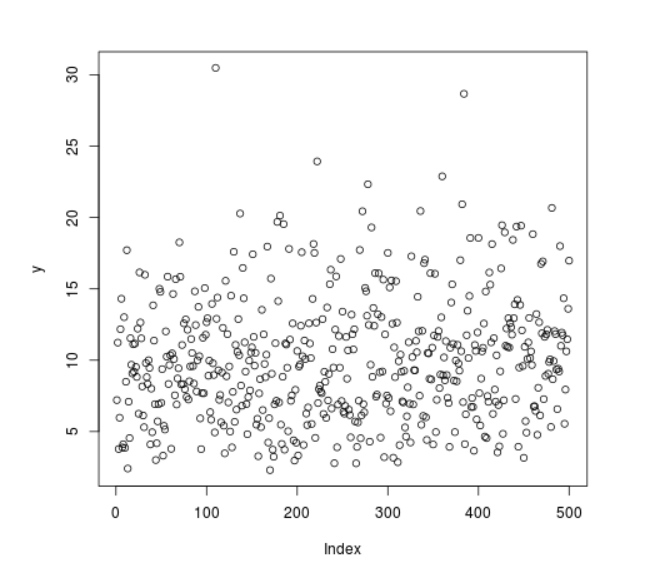# Compute Randomly Drawn Chi Square Density in R Programming – rchisq() Function

`rchisq()` function in R Language is used to compute random chi square density for a set of values.

Syntax: rchisq(N, df)

Parameters:
N: Sample Size
df: Degree of Freedom

Example 1:

 `# R program to generate  ` `# random chi square density points ` ` `  `# Setting seed for ` `# random number generation ` `set``.seed(``1000``) ` ` `  `# Set sample size ` `N <``-` `20` ` `  `# Calling rchisq() Function ` `y <``-` `rchisq(N, df ``=` `10``) ` `y  `

Output:

```   7.208082 11.236799  3.780669  5.972266 12.174243 14.308333  3.886482
  4.117081 13.015581  3.864009  8.494487 17.713528  2.411329  7.096494
  4.546996 11.542960  9.683502  9.073933 11.120782  9.193626
```

Example 2:

 `# R program to generate  ` `# random chi square density points ` ` `  `# Setting seed for ` `# random number generation ` `set``.seed(``1000``) ` ` `  `# Set sample size ` `N <``-` `500` ` `  `# Calling rchisq() Function ` `y <``-` `rchisq(N, df ``=` `10``) ` ` `  `# Plotting graph ` `plot(y) `

Output:My Personal Notes arrow_drop_upCheck out this Author's contributed articles.

If you like GeeksforGeeks and would like to contribute, you can also write an article using contribute.geeksforgeeks.org or mail your article to contribute@geeksforgeeks.org. See your article appearing on the GeeksforGeeks main page and help other Geeks.

Please Improve this article if you find anything incorrect by clicking on the "Improve Article" button below.

Article Tags :

Be the First to upvote.

Please write to us at contribute@geeksforgeeks.org to report any issue with the above content.